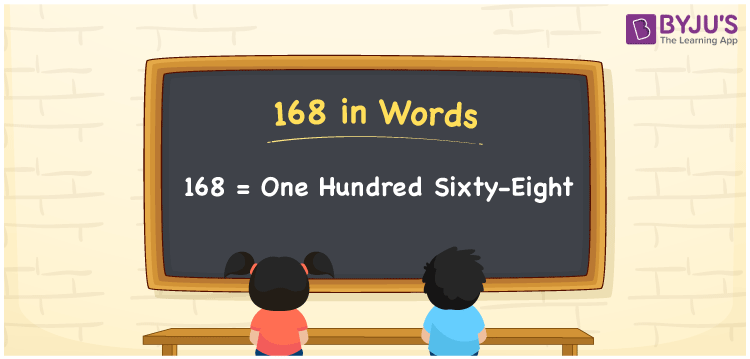# 168 in Words

168 in words is written as One hundred sixty-eight. In both the International System of Numerals and the Indian System of Numerals, 168 is written as One hundred sixty-eight. The number 168 is a Cardinal Number as it represents some quantity. For example, “there are 168 chairs in this room”.

 168 in Words One hundred sixty-eight One hundred sixty-eight in Number 168

## 168 in English Words

We write 168 in English Words using the letters of the English alphabet. Therefore, we read 168 in English as “One hundred sixty-eight.”## How to Write 168 in Words?

To write 168 in words, we shall use the place value chart. In the place value chart, write 1 in the hundreds, 6 in the tens, and 8 in the ones, respectively. Now let us make a place value chart to write the number 168 in words.

 Hundreds Tens Ones 1 6 8

Thus, we can write the expanded form as

1 × Hundred + 6 × Ten + 8 × One

= 1 × 100 + 6 × 10 + 8 × 1

= 100 + 60 + 8

= 168

= One hundred sixty-eight.

168 is a natural number, the successor of 167 and the predecessor of 169.

168 in words – One hundred sixty-eight

• Is 168 an odd number? – No
• Is 168 an even number? – Yes
• Is 168 a perfect square number? – No
• Is 168 a perfect cube number? – No
• Is 168 a prime number? – No
• Is 168 a composite number? – Yes

## Frequently Asked Questions on 168 in Words

Q1

### How to write 168 in words?

168 in words is written as One hundred sixty-eight.
Q2

### How to write 168 in the International and Indian System of Numerals?

In both, the system of numerals, 168 in words, is written as One hundred sixty-eight.
Q3

### What are the three consecutive natural numbers after 168?

The three consecutive natural numbers after 168 are 169, 170 and 171.